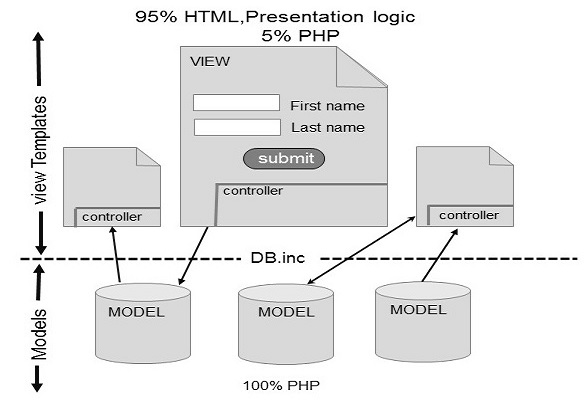# PHP - Design Patterns

Microsoft design pattern Theory is, "The document introduces patterns and then presents them in a repository, or catalogue, which is organized to help you locate the right combination of patterns that solves your problem".

## Examples of Design patterns

### Singleton

A Class has one instance, It provides a global access point to it, Following code will explain about singleton concept.

```<?php
class Singleton {
public static function getInstance() {
static \$instance = null;

if (null === \$instance) {
\$instance = new static();
}
return \$instance;
}
protected function __construct() {
}

private function __clone() {
}

private function __wakeup() {
}
}

class SingletonChild extends Singleton {
}

\$obj = Singleton::getInstance();
var_dump(\$obj === Singleton::getInstance());

\$anotherObj = SingletonChild::getInstance();
var_dump(\$anotherObj === Singleton::getInstance());
var_dump(\$anotherObj === SingletonChild::getInstance());
?>
```

Above Example implemented based on static method creation is getInstance()

### Factory

A Class Simple Creates the object and you want to use that object, Following example will explain about factory design pattern.

```<?php
class Automobile {
private \$bikeMake;
private \$bikeModel;

public function __construct(\$make, \$model) {
\$this->bikeMake = \$make;
\$this->bikeModel = \$model;
}

public function getMakeAndModel() {
return \$this->bikeMake . ' ' . \$this->bikeModel;
}
}

class AutomobileFactory {
public static function create(\$make, \$model) {
return new Automobile(\$make, \$model);
}
}

\$pulsar = AutomobileFactory::create('ktm', 'Pulsar');
print_r(\$pulsar->getMakeAndModel());

class Automobile {
private \$bikeMake;
private \$bikeModel;

public function __construct(\$make, \$model) {
\$this->bikeMake = \$make;
\$this->bikeModel = \$model;
}

public function getMakeAndModel() {
return \$this->bikeMake . ' ' . \$this->bikeModel;
}
}

class AutomobileFactory {
public static function create(\$make, \$model) {
return new Automobile(\$make, \$model);
}
}
t\$pulsar = AutomobileFactory::create('ktm', 'pulsar');

print_r(\$pulsar->getMakeAndModel());
?>
```

The main difficulty with factory pattern is it will increase the complexity and it is not reliable for good programmers.

### Strategy pattern

Strategy pattern makes a family algorithm and encapsulates each algorithm. Here each algorithm should be inter-changeable within the family.

```<?php
\$elements = array(
array(
'id' => 2,
'date' => '2011-01-01',
),
array(
'id' => 1,
'date' => '2011-02-01'
)
);

\$collection = new ObjectCollection(\$elements);

\$collection->setComparator(new IdComparator());
\$collection->sort();

echo "Sorted by ID:\n";
print_r(\$collection->elements);

\$collection->setComparator(new DateComparator());
\$collection->sort();

echo "Sorted by date:\n";
print_r(\$collection->elements);
?>
```

## Model View Control

The View acts as GUI, Model Acts as Back End and Control acts as an adapter. Here three parts are interconnected with each other. It will pass the data and access the data between each other.